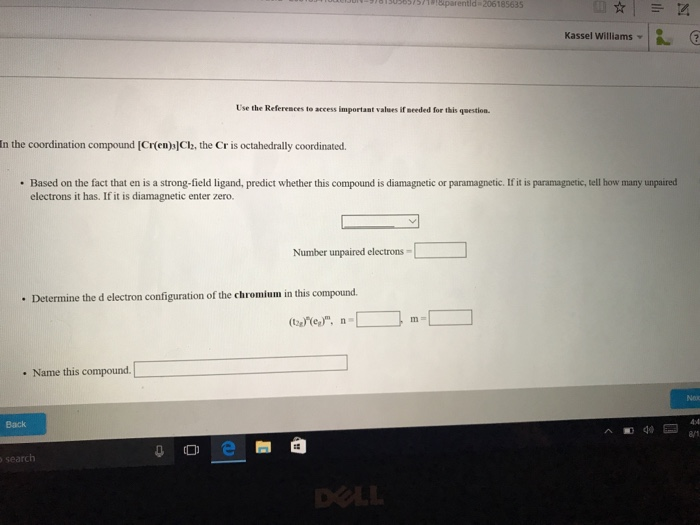# Question & Answer: In the coordination compound [Cr(en)_3]Cl_2, the Cr is octahedrally coordinated. Based on…..In the coordination compound [Cr(en)_3]Cl_2, the Cr is octahedrally coordinated. Based on the fact that en is a strong-field ligand, predict whether this compound is diamagnetic or paramagnetic. If it is paramagnetic, tell how many unpaired electrons it has. If it is diamagnetic enter zero. Number unpaired electrons = Determine the d electron configuration of the chromium in this compound. (t_2 g)^n (e_g)^m, n = m = Name this compound.